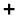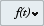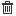# Define Multiplier Functions

Use the Multipliers tool to create, edit, and delete multiplier functions. Multiplier or ramp functions are used to multiply or ramp variables while defining boundaries, radiation, and mesh conditions.

## Create Multiplier Functions

Use the Multipliers tool to create new multiplier functions.

1. From the Flow ribbon, click the arrow next to the Setup tool set, then select Multipliers.
The Multiplier Library opens.
2. Clickto add a new multiplier.
A multiplier creation dialog opens.
3. Select a constant or varying multiplier type and input values.
Option Description
Constant Define a constant multiplying value.
Piecewise linear, Piecewise Log Linear, Cubic Spline Use the table and the plot area.

You can use existing pre-defined functions to populate the data then edit the data using the table or interactively with the plot.

Sinusoidal Define frequency, amplitude, and phase.
User Function Enter a function then select an argument type from the drop-down menu and input values in the table.
4. Select a Variable, Evaluation method, and Filter.
5. Close the dialog.
Once multipliers are defined, they can be applied to domains, boundaries, radiation, or motion definitions. Clickin a dialog to select a multiplier. You can also have the option to create a new multiplier.

## Edit Multiplier Functions

Use the Multipliers tool to edit existing multiplier functions.

1. From the Flow ribbon, click the arrow next to the Setup tool set, then select Multipliers.
The Multiplier Library opens
2. Right-click on a multiplier and select Edit.
A multiplier creation dialog opens.
3. Optional: Click the name in the top-left corner of the dialog to rename the multiplier.
This can also be done by double-clicking on the Name field in the Multiplier Library.
4. Edit the multiplier parameters.
3. Clickor right-click and select Delete.